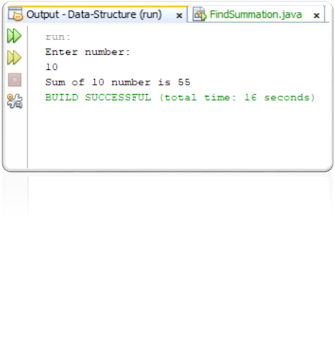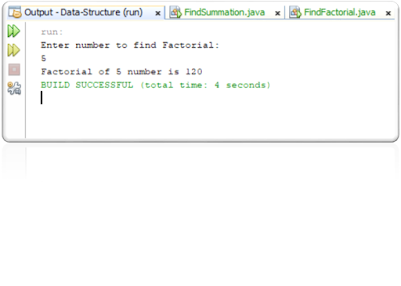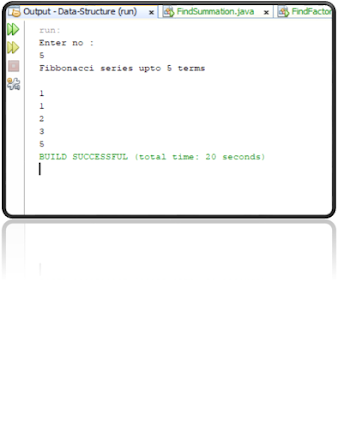# Top 3 recursive function problem in java

TOP 3 RECURSIVE FUNCTION PROBLEM IN JAVA.

Welcome to Nowtechspot, in this article, we are going to know that the Top 3 recursive function problem in java. Before reading this article you should have to know the basic or fundamental concept of java programming language.recursive function problem in java

What is meant by recursion?

In short, a function calling itself, it’s a recursion.

video.

These concepts:

·

·

·

·

·       Static keyword.

·       Printing on console.

A short note explanation of “What I have used to write code“.

·       I have used 3 different Class for 3 different program

1.      FindSummation.

2.    FindFactorial.

3.    FibClass.

Important Questions are related to this recursion problem that helps you not to worry about java statements that are written into the code.

Questions.

recursive function problem in java related questions.

1.      What the Scanner Class can do?

2.    Why we pass System.in is an Argument?

3.    Why static method we required in all 3 of these codes?

4.   Why we import java.util.Scanner?

If you already knew this silly problem then go further and check out the code of it.

Answers.

1. and 2. Scanner class can be used to take input from the user with the help of System.in object.

Generally when user type any character and number that can stores into the buffer and System.in is responsible for adding and fetching data to the buffer and from the buffer respectively.

·       Scanner class is the final class that cannot be extended.

·       Scanner class is part of the java.util package.

USEFUL METHODS.

1.      next( ).

2.    nextLine( ).

3.    nextBoolean ( ).

4.   nextByte( ).

5.    nextDouble( ).

6.    nextFloat( ).

7.    nextInt( ).

8.   nextLong( ).

9.    nextShort( ).

3. Because main () method is a static method and every method which have been called from inside the main ( ) body that should be static. That’s why we use static keyword.

4. Because the Scanner class are present in these package.

Note: Code is written into NetBeans.

1.   Write a program to find the summation of “n” number using recursion.

recursive function problem in java

·       CODE.

/* Program to write code to find Summation of n numbers using recursively */

import java.util.Scanner;

public class FindSummation {

public static void main(String[] args) {

Scanner sc = new Scanner(System.in);

System.out.println("Enter number: ");

int n = sc.nextInt();

System.out.println("Sum of "+n+" number is "+sum(n));

}

public static int sum(int n){

int s = 0;

if (n==1){

return n;

}

s = n + sum(n-1);

return s;

}

}

·       OUTPUT.recursive function problem in java

1.   Write a program to find the factorial of “n” number using recursion.

·       CODE.

/* write a program to find Factorial of n number */

import java.util.Scanner;

import java.util.InputMismatchException;

import java.lang.RuntimeException;

public class FindFactorial {

public static int n;

public static void main(String[] args){

System.out.println("Enter number to find Factorial: ");

Scanner sc = new Scanner(System.in);

try{

n = sc.nextInt();

}

catch(InputMismatchException e){

System.out.println("Exception is "+e.getMessage());

}

finally{

System.out.println("Factorial of "+n+" number is "+fact(n));

}

}

public static int fact(int n){

int fact = 1;

if(n == 1){

return 1;

}

fact = n * fact(n-1);

return fact;

}

}

·       OUTPUT.recursive function problem in java

1.   Write a program to find the Fibonacci series up to “n” terms using recursion.

·       CODE.

import java.util.Scanner;

public class FibClass {

public static void main(String[] args) {

Scanner sc = new Scanner(System.in);

System.out.println("Enter no :");

int n = sc.nextInt();

System.out.println("Fibbonacci series upto "+n+" terms\n");

for(int i=1;i<=n;i++)

System.out.println(fib(i));

}

public static int fib(int n){

if(n==1 || n==2)

return 1;

return (fib(n-1) + fib(n-2));

}

}

·       OUTPUT.recursive function problem in java

Internal linking.

social links:

recent post:

If you like this article and if it is informative or helpful for you. please let me know in the comment section.